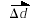#### Units and Standards

1. Units and Standards

There are 4 fundamental scientific measurements: Mass, Length, Time, and Electric Charge

The following table summarizes their basic SI (International Metric System) units

 Fundamental Quantity Mass Length, distance, displacement Time Charge SI Unit kg (kilogram) m (metre) s (second) C (coulomb) Symbol m Δd t Q

2. Scalar vs. Vector

Scalar quantities express only a quantity and a unit

Vector quantities express a quantity, a unit and a direction

Examples:

 Quantity Symbol Type Example distance Δd scalar Δd = 10 m displacementvector= 10 m [E]

Note the arrow on the symbol for a vector.
It symbolizes the direction property of the vectory.